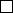3Linear maps

IA Vectors and Matrices3.2 Linear Maps
Definition
(Domain, codomain and image of map)
.
Consider sets
A
and
B
and mapping
T
:
A B
such that each
x A
is mapped into a unique
x
0
=
T
(
x
)
B
.
A
is the domain of
T
and
B
is the co-domain of
T
. Typically,
we have T : R
n
R
m
or T : C
n
C
m
.
Definition
(Linear map)
.
Let
V, W
be real (or complex) vector spaces, and
T : V W . Then T is a linear map if
(i) T (a + b) = T (a) + T (b) for all a, b V .
(ii) T (λa) = λT (a) for all λ R (or C).
Equivalently, we have T (λa + µb) = λT (a) + µT (b).
Example.
(i)
Consider a translation
T
:
R
3
R
3
with
T
(
x
) =
x + a
for some fixed,
given
a
. This is not a linear map since
T
(
λx
+
µy
)
6
=
λx
+
µy
+ (
λ
+
µ
)
a
.
(ii) Rotation, reflection and projection are linear transformations.
Definition
(Image and kernel of map)
.
The image of a map
f
:
U V
is the
subset of V {f(u) : u U}. The kernel is the subset of U {u U : f (u) = 0}.
Example.
(i)
Consider
S
:
R
3
R
2
with
S
(
x, y, z
) = (
x
+
y,
2
x z
). Simple yet
tedious algebra shows that this is linear. Now consider the effect of
S
on
the standard basis.
S
(1
,
0
,
0) = (1
,
2),
S
(0
,
1
,
0) = (1
,
0) and
S
(0
,
0
,
1) =
(0
,
1). Clearly these are linearly dependent, but they do span the whole
of R
2
. We can say S(R
3
) = R
2
. So the image is R
2
.
Now solve
S
(
x, y, z
) =
0
. We need
x
+
y
= 0 and 2
x z
= 0. Thus
x
=
(
x, x,
2
x
), i.e. it is parallel to (1
,
1
,
2). So the set
{λ
(1
,
1
,
2) :
λ R}
is the kernel of S.
(ii)
Consider a rotation in
R
3
. The kernel is the zero vector and the image is
R
3
.
(iii)
Consider a projection of
x
onto a plane with normal
ˆn
. The image is the
plane itself, and the kernel is any vector parallel to ˆn
Theorem.
Consider a linear map
f
:
U V
, where
U, V
are vector spaces.
Then im(f) is a subspace of V , and ker(f) is a subspace of U .
Proof. Both are non-empty since f(0) = 0.
If
x, y im
(
f
), then
a, b U
such that
x
=
f
(
a
)
, y
=
f
(
b
). Then
λx
+
µy
=
λf
(
a
) +
µf
(
b
) =
f
(
λa
+
µb
). Now
λa
+
µb U
since
U
is a vector
space, so there is an element in
U
that maps to
λx
+
µy
. So
λx
+
µy im
(
f
)
and im(f) is a subspace of V .
Suppose
x, y ker
(
f
), i.e.
f
(
x
) =
f
(
y
) =
0
. Then
f
(
λx
+
µy
) =
λf
(
x
) +
µf(y) = λ0 + µ0 = 0. Therefore λx + µy ker(f).